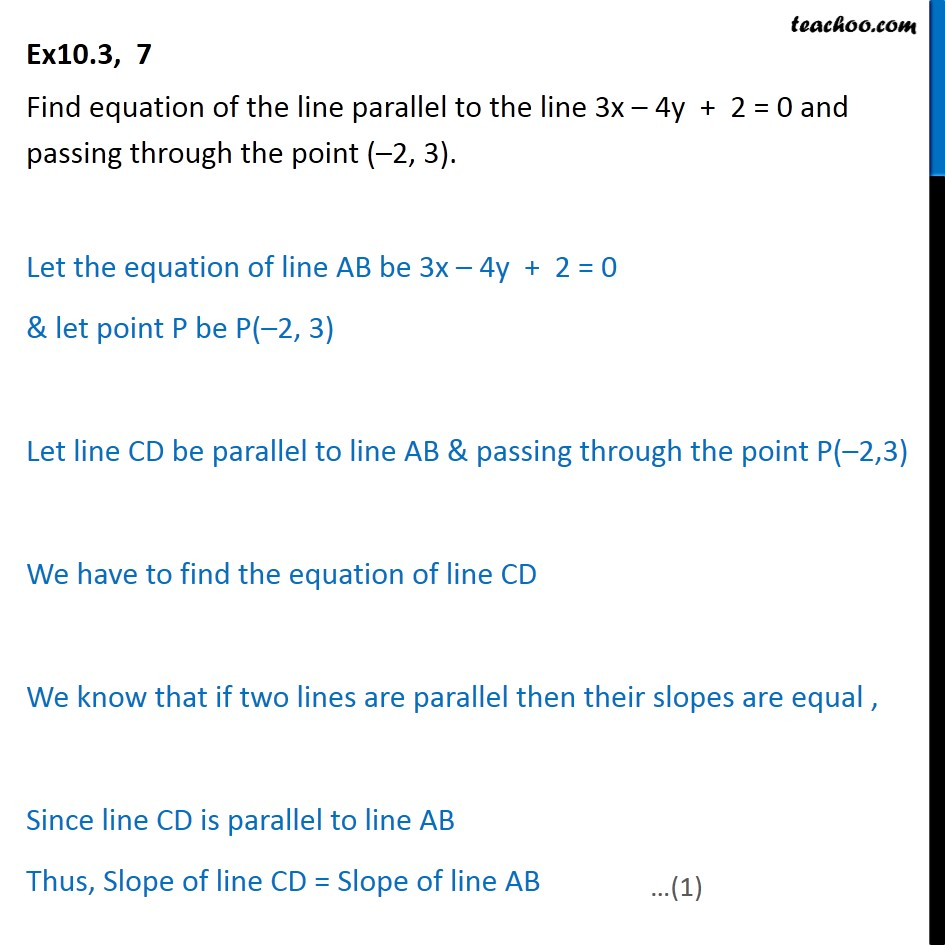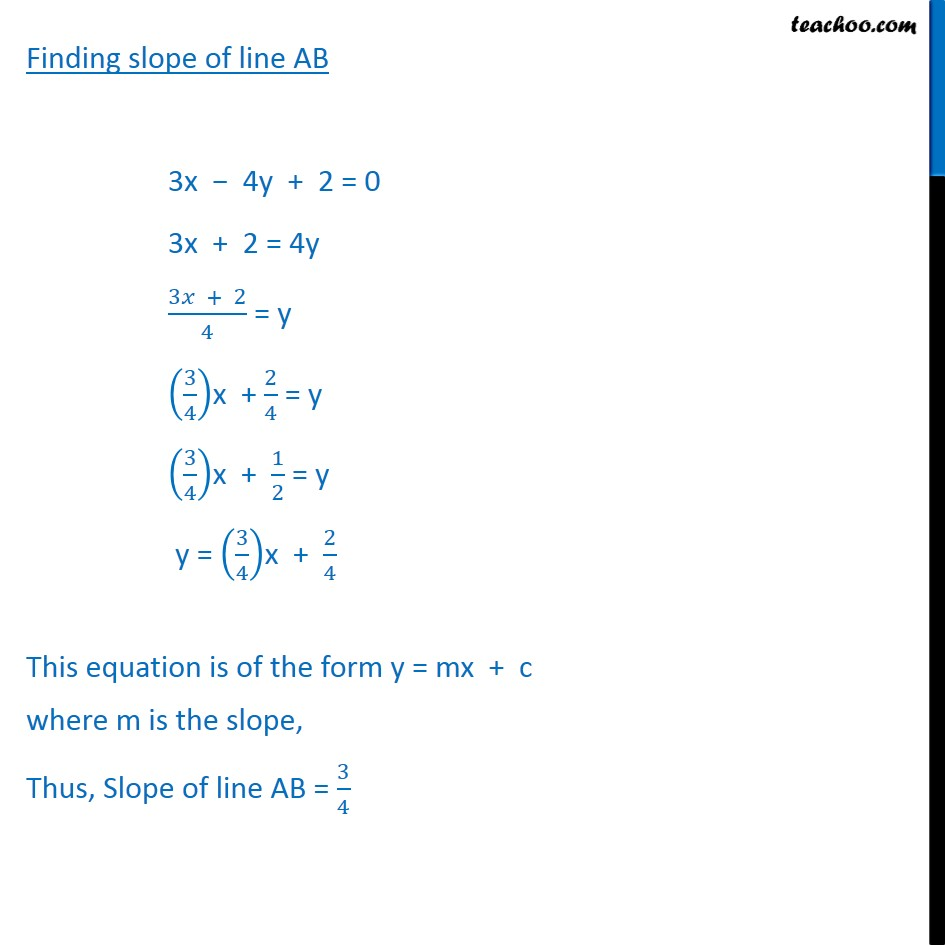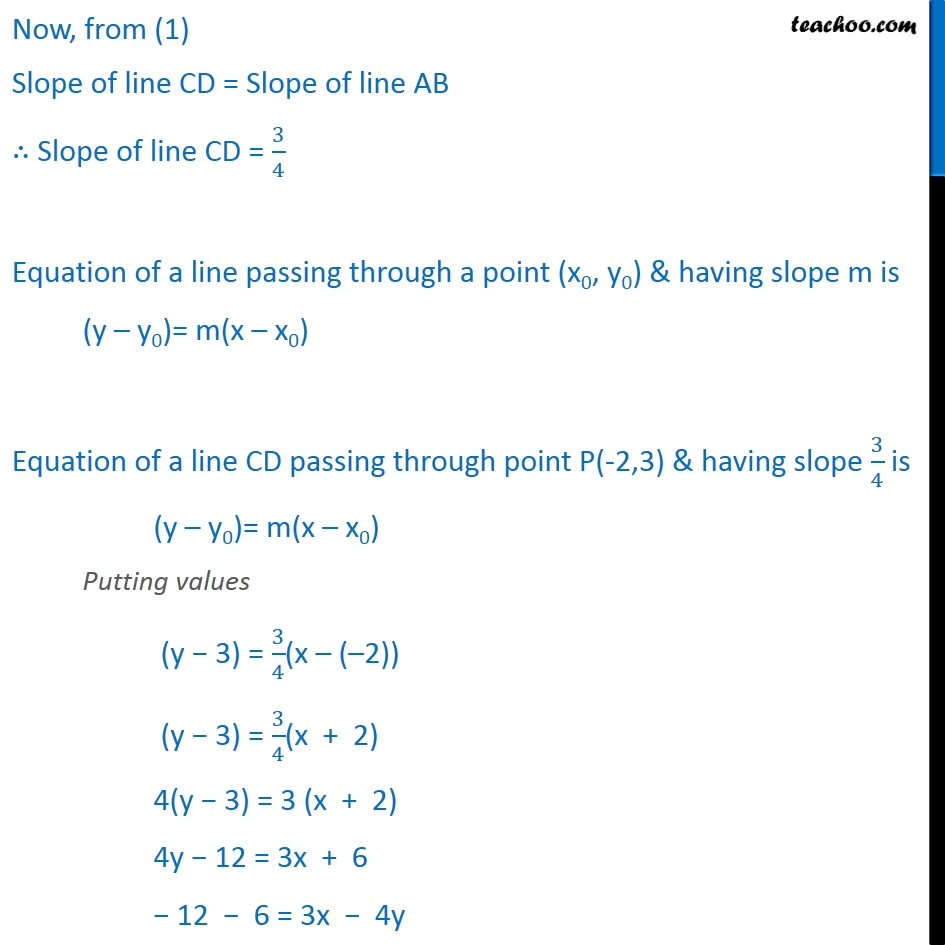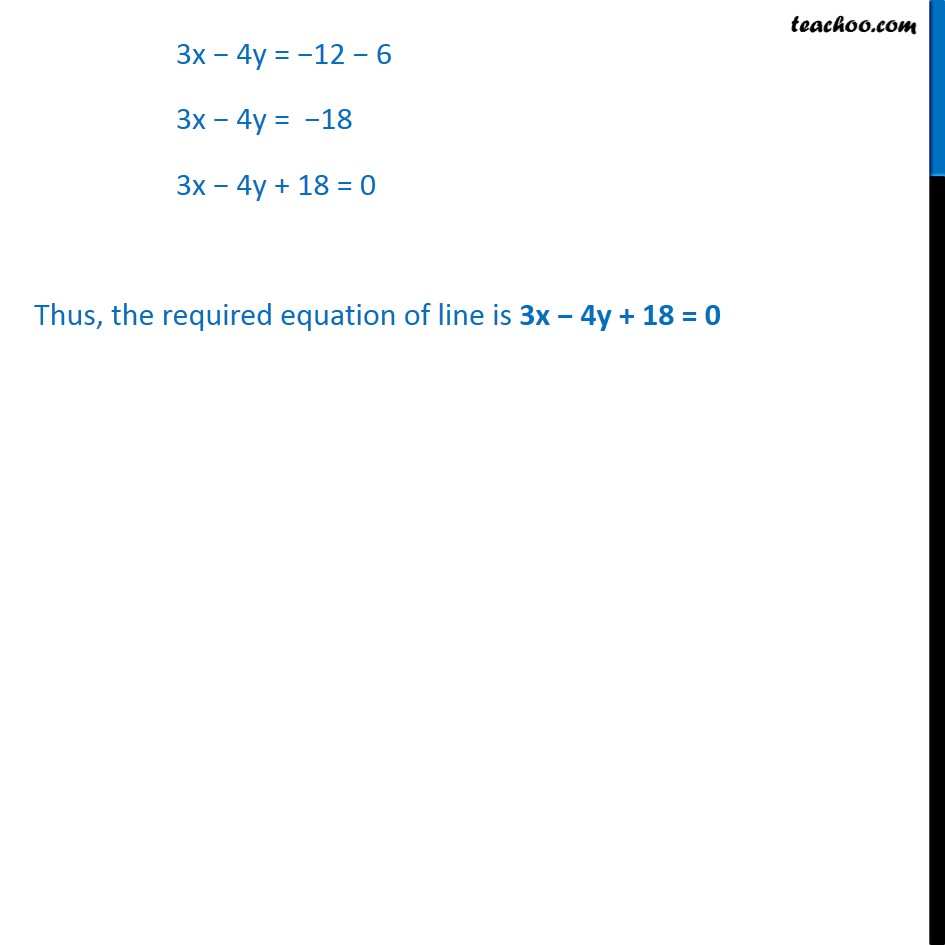Ex 9.3

Chapter 9 Class 11 Straight Lines
Serial order wiseLearn in your speed, with individual attention - Teachoo Maths 1-on-1 Class

### Transcript

Ex10.3, 7 Find equation of the line parallel to the line 3x – 4y + 2 = 0 and passing through the point (–2, 3). Let the equation of line AB be 3x – 4y + 2 = 0 & let point P be P(–2, 3) Let line CD be parallel to line AB & passing through the point P(–2,3) We have to find the equation of line CD We know that if two lines are parallel then their slopes are equal , Since line CD is parallel to line AB Thus, Slope of line CD = Slope of line AB Finding slope of line AB 3x − 4y + 2 = 0 3x + 2 = 4y (3𝑥 + 2)/4 = y (3/4)x + 2/4 = y (3/4)x + 1/2 = y y = (3/4)x + 2/4 This equation is of the form y = mx + c where m is the slope, Thus, Slope of line AB = 3/4 Now, from (1) Slope of line CD = Slope of line AB ∴ Slope of line CD = 3/4 Equation of a line passing through a point (x0, y0) & having slope m is (y – y0)= m(x – x0) Equation of a line CD passing through point P(-2,3) & having slope 3/4 is (y – y0)= m(x – x0) Putting values (y − 3) = 3/4(x – (–2)) (y − 3) = 3/4(x + 2) 4(y − 3) = 3 (x + 2) 4y − 12 = 3x + 6 − 12 − 6 = 3x − 4y 3x − 4y = − 12 − 6 3x − 4y = − 18 3x + 4y + 18 = 0 Thus, the required equation of line is 3x + 4y + 18 = 0